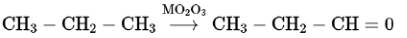# Which of the following reagent is used for the following reaction?

Question:

Which of the following reagent is used for the following reaction?

$\mathrm{CH}_{3} \mathrm{CH}_{2} \mathrm{CH}_{3} \stackrel{?}{\longrightarrow} \mathrm{CH}_{3} \mathrm{CH}_{2} \mathrm{CHO}$

1. Manganese acetate

2. Copper at high temperature and pressure

3. Molybdenum oxide

4. Potassium permanganate

Correct Option: , 2

Solution: